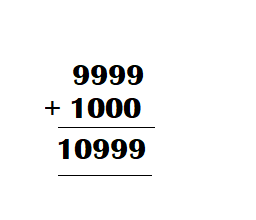Challenging Practice

Use the digits below. Make as many 4-digit numbers as you can. Do not begin with ‘0’.
For each number, use each digit only once.
Then add two 4-digit numbers where you do not need to regroup.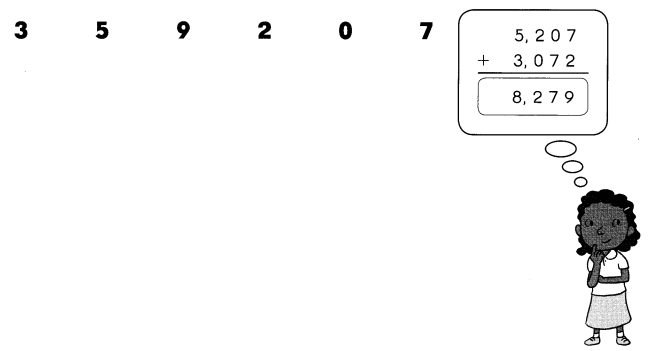Question 1.
Now you try it!
2350 + 7023 = 9373.
3207 + 2570 = 5777.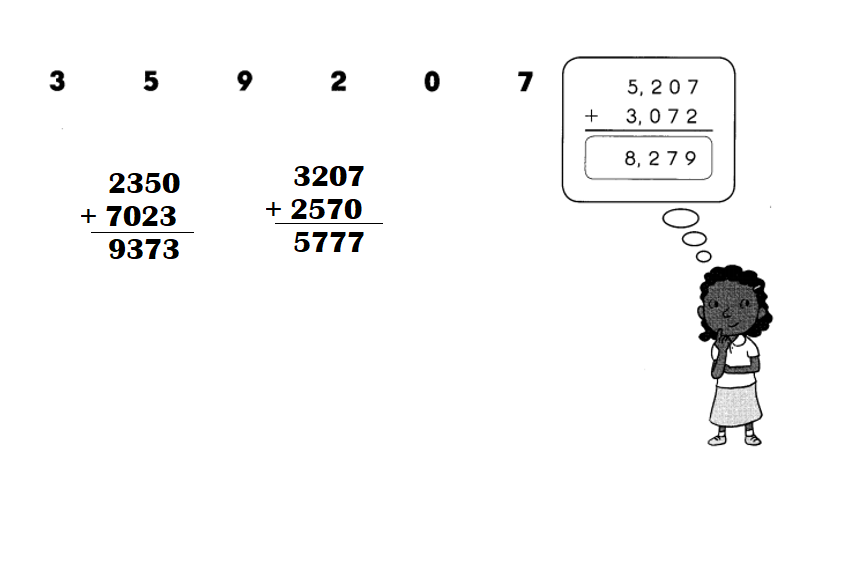Explanation:
Numbers to use to make 4 digit numbers:
3 5 9 2 0 7
3592
5920
9207
2350
5320
7023
3207
2570

Question 2.
Use the digits below. Make as many 4-digit numbers as you can.
Do not begin with ‘0’.
For each number, use each digit only once.
Then add two 4-digit numbers where you need to regroup.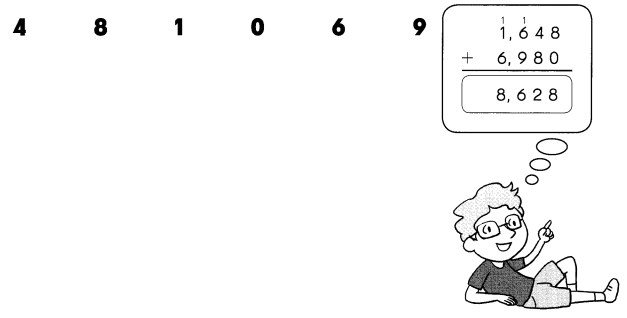4810 + 1069 = 5879.
1048 + 4801 = 5849.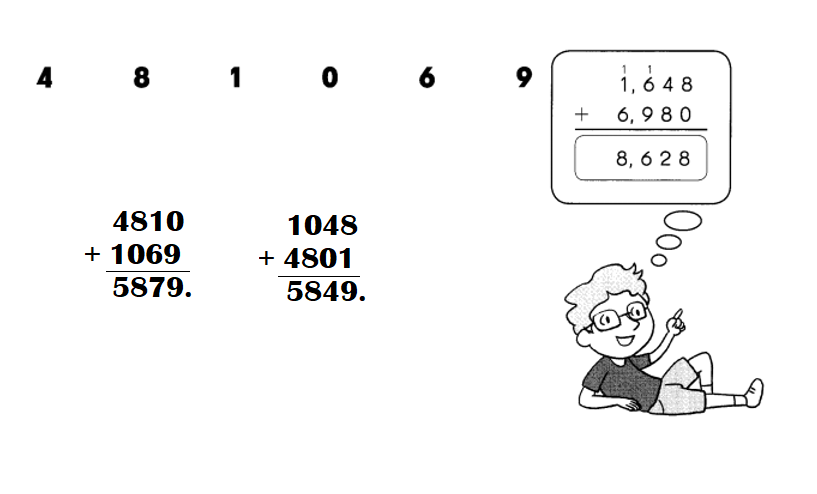Explanation:
Numbers to use to make 4 digit numbers:
4 8 1 0 6 9
4810
8106
1069
1048
6109
8104
8401
8604
9810
9601
9840

Find the missing numbers.
Question 1.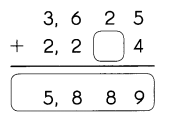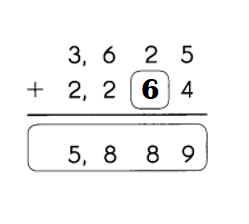Explanation:
Missing number:
2 + ? = 8
=> ? = 8 – 2
=> ? = 6.

Question 2.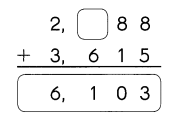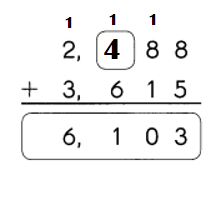Explanation:
Missing number:
Ones place number = 8 + 5 = 13.
Tens place number = 1 + 8 + 1 = 10.
Hundreds place number = 1 + ? + 6 = 11.
=> 7 + ? = 11
=> ? = 11 – 7
=> ? = 4.
Thousands place number = 1 + 2 + 3 = 6.

Find the page numbers of the book.
Question 3.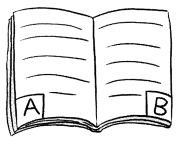Each of the pages has a 3-digit page number.
The number on Page A is an even number.
The sum of its digits is 7.
The number on Page B is an odd number.
The sum of its digits is 8.
What are the two possible page numbers for Page A and Page B?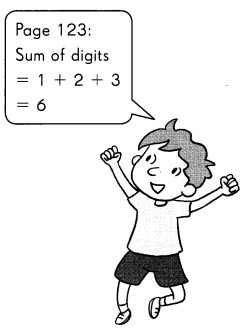Number on page A:
? + ? + ? = 7.
=> 2 + 1 + 4 = 7.
=> 1 + 3 + 3 = 7.
Number on page B:
? + ? + ? = 8.
=> 2 + 1 + 5 = 8.
=> 1 + 3 + 4 = 8.

Explanation:
Each of the pages has a 3-digit page number.
The number on Page A is an even number.
The sum of its digits is 7.
Number on page A:
? + ? + ? = 7.
=> 2 + 1 + 4 = 7.
=> 1 + 3 + 3 = 7.
Each of the pages has a 3-digit page number.
The number on Page B is an odd number.
The sum of its digits is 8.
Number on page B:
? + ? + ? = 8.
=> 2 + 1 + 5 = 8.
=> 1 + 3 + 4 = 8.

Solve.
Question 4.
Find two numbers whose sum is 100.
_____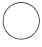____ = 100
Two numbers whose sum is 100:
53 + 47 = 100.

Explanation:
Two numbers whose sum is 100.
Let the first number be 53.
Second number = 53 + ?? = 100.
=> ?? = 100 – 53
=> ?? = 47.
53 + 47 = 100.

Question 5.
Find three numbers whose sum is 150.
___________ = 150
Three numbers whose sum is 150:
24 + 31 + 95 = 150.

Explanation:
Three numbers whose sum is 150:
Let the first number be 24.
Second number be 31.
Third number = 24 + 31 + ?? = 150.
=> 55 + ?? = 150.
=> ?? = 150 – 55
=> ?? = 95.
24 + 31 + 95 = 150.

Question 6.
A student has four digits.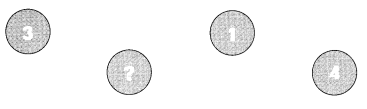The digit in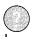is greater than each of the other digits but is less than the sum of these digits.
What is the greatest possible digit?
The greatest possible digit can be 5 or 6 or 7 because it is greater than 1, 3, 4 other numbers.

Explanation:
A student has four digits:
3 ? 1 4
The digit inis greater than each of the other digits but is less than the sum of these digits.
Sum of digits = 3 + 1 + 4 = 4 + 4 = 8.
The greatest possible digit can be 5 or 6 or 7 because it is greater than 1, 3, 4 other numbers.

Use the given digits and the answer you found in Exercise 6 to answer Exercises 7 to 9.
Question 7.
What is the greatest possible 4-digit number?
9999 is the greatest possible 4-digit number.

Explanation:
The greatest possible 4-digit number:
9999.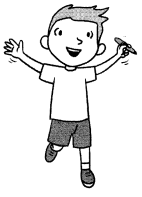Question 8.
What is the least possible 4-digit number?
1000 is the least possible 4-digit number.

Explanation:
The least possible 4-digit number is 1000.

Question 9.
What is the sum of the 4-digit numbers in Exercises 7 and 8?